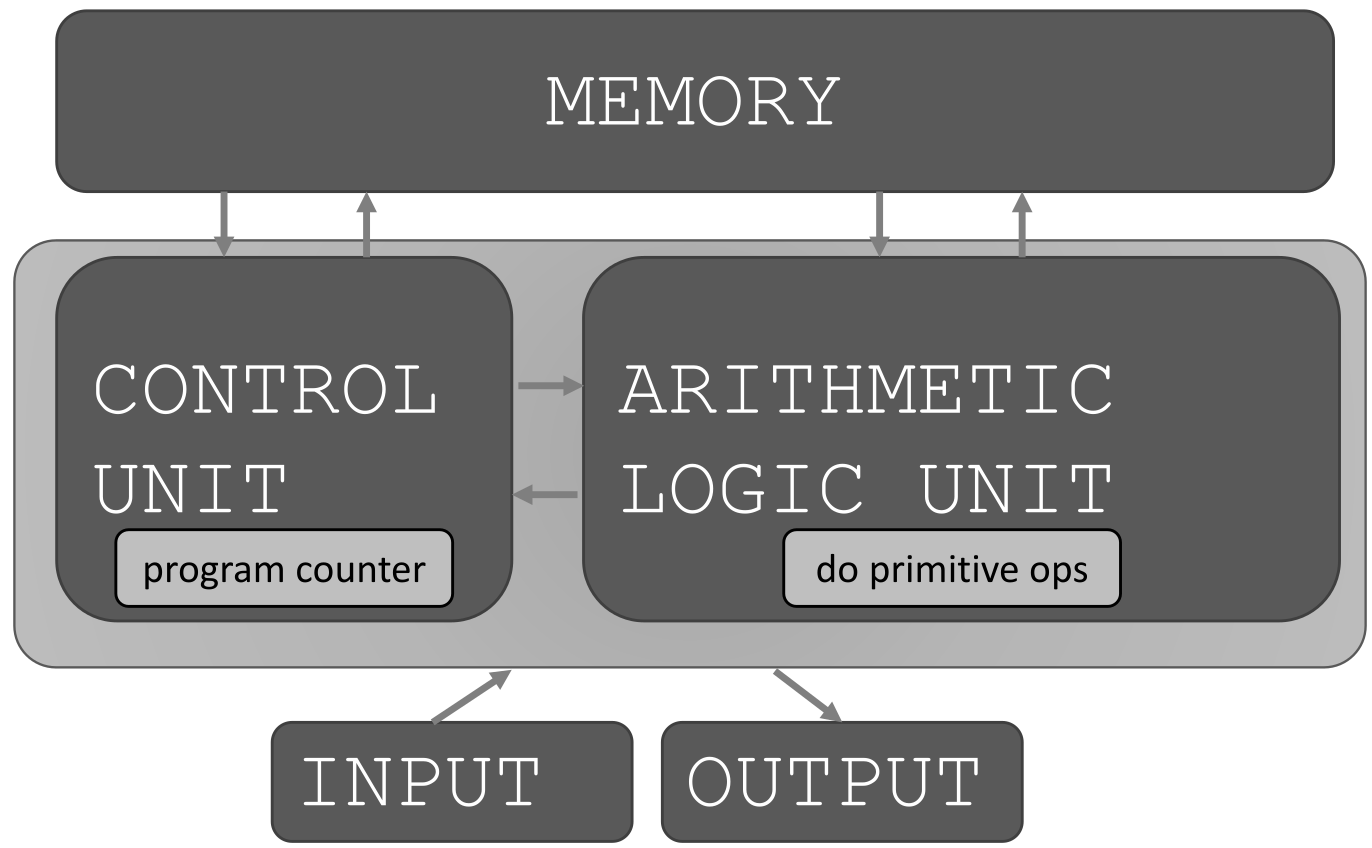# Lecture 1

## Welcome to MUH101

Burkay Genç, Ahmet Selman Bozkır, and Selma Dilek

01/03/2023

## Today

• course Info
• what is computation
• python basics
• mathematical operations
• python variables and types

## Course Info

• Subject : Learn Python a programming language
• Classes :
• All classes are online until further notice
• All off-class communication will be on hadi

## Course Info

• 60%: Midterm (2x)
• Midterms format will be announced during the semester
• 40%: Final Exam (1x)

## Course Info

• You are strictly forbidden to cooperate on exams
• You are strictly forbidden to search for answers on the Internet
• You are strictly forbidden to copy/paste materials from the Internet

## COURSE POLICIES

• Cooperation
• you may cooperate with your friends to understand the course material
• all exam work needs to be individual
• cheaters will be harshly penalized -> -100, F3, disciplinary committee

## FAST PACED COURSE

• Position yourself to succeed!
• do practice early
• do not skip lectures
• New to programming? PRACTICE. PRACTICE? PRACTICE!
• can’t passively absorb programming as a skill
• don’t be afraid to try out Python commands!

## TOPICS

• represent knowledge with data structures
• iteration and recursion as computational metaphors
• abstraction of procedures and data types
• organize and modularize systems using object classes and methods
• [maybe] different classes of algorithms, searching and sorting
• [maybe] complexity of algorithms

## PYTHON IDE

• What is an IDE?
• An Integrated Development Environment allows you to write programs in a programming language and provides extra tools to help the process
• Syntax highlighting
• Code completion
• Bracket completion/matching
• Debugging
• Profiling
• more…
• PyCharm is a very powerful and popular IDE for Python
• IDLE comes built-in with any Python distribution
• Eric, Spyder, Eclipse+PyDev
• Sublime Text, VS Code, Atom, etc. are generic editors that can be extended with Python capabilities

## WHAT DOES A COMPUTER DO

• Fundamentally:
• performs calculations
a billion calculations per second!
• remembers results
100s of gigabytes of storage!
• What kinds of calculations?
• built-in to the language
• ones that you define as the programmer
• computers only know what you tell them

## TYPES OF KNOWLEDGE

• declarative knowledge is statements of fact.

• someone will win a Trophy before class ends
• imperative knowledge is a recipe or “how-to”.

2. Burkay opens his IDE
3. Burkay chooses a random number between 1st and nth responder
4. Burkay finds the number in the responders sheet. Winner!

## A NUMERICAL EXAMPLE

• square root of a number $x$$x$ is $y$$y$ such that $y\ast y=x$$y*y = x$

• recipe for deducing square root of a number $x$$x$

1. Start with a guess, $g$$g$
2. If $g\ast g$$g*g$ is close enough to $x$$x$, stop and say $g$$g$ is the answer
3. Otherwise make a new guess by averaging $g$$g$ and $x/g$$x/g$
4. Using the new guess, repeat process until close enough
$g$$g$ $g\ast g$$g*g$ $x/g$$x/g$ $\left(g+x/g\right)/2$$(g+x/g)/2$
3 9 16/3 4.17
4.17 17.36 3.837 4.0035
4.0035 16.0277 3.997 4.000002

## WHAT IS A RECIPE

1. sequence of simple steps
2. flow of control process that specifies when each step is executed
3. a means of determining when to stop

1 + 2 + 3 = an algorithm!

## COMPUTERS ARE MACHINES

• how to capture a recipe in a mechanical process
• fixed program computer
• calculator
• stored program computer
• machine stores and executes instructions

## BASIC MACHINE ARCHITECTUREBasic machine architecture

## STORED PROGRAM COMPUTER

• sequence of instructions stored inside computer
• built from predefined set of primitive instructions
• arithmetic and logic
• simple tests
• moving data
• special program (interpreter) executes each instruction in order
• use tests to change flow of control through sequence
• stop when done

## BASIC PRIMITIVES

• Turing showed that you can compute anything using 6 primitives
• modern programming languages have more convenient set of primitives
• we can also create new primitives
• anything computable in one language is computable in any other programming language
• Python == Java == C == Pascal == C++ == C#
• They only differ in ease of doing something

## CREATING RECIPES

• a programming language provides a set of primitive constructs
• 2, 4.8, ‘a’, “burkay”, TRUE, +, -, *, …
• expressions are complex but legal combinations of primitives in a programming language
• 2 + 4.7
• TRUE && FALSE
• 3.0 + 2 / 5.4
• expressions and computations have values and meanings in a programming language

## ASPECTS OF LANGUAGES

• primitive constructs
• English: words
• programming language: numbers, strings, simple operators